## Calculation of lattice cookie density

To compare the ideal efficiency of different arrangements, we can look at each lattice’s representative “unit cell” — i.e., the shape that is repeated in the pattern:In a square lattice, each of the squares holds four cookie quarters, one in each corner, for a total of one cookie. The square takes up an area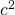, whereis the distance between cookie centers. So the “cookie density” is:

(1 cookie per square)/(area of the square) =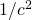Each triangle in the triangular lattice, meanwhile, holds three sixths of a cookie (for a total of one half cookie) and takes up area (1/2)(base)(height) =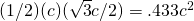. So its cookie density is:

(1/2 cookie per triangle)/(area of the triangle) =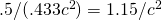Alternately, to calculate what percent of the area is taken up by cookies (rather than the space between them), divide the area of cookie in the unit cell by the total unit cell area. (This is equivalent to multiplying the area of a cookie by the cookie density.) When the cookies are touching, the radius of a cookie equals c/2, so the area is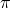(radius)=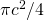.

square unit cell: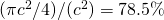triangular unit cell: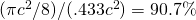Those are the theoretical values. When you have boundaries, however, the cookie density at the edges is lower due to there being extra space there (but not enough extra space to fit another cookie). The only time the density reaches the theoretical value is for a square lattice on a cookie sheet that’s an exact number of cookies in both dimensions. Here is a graph showing how cookie coverage depends on width, when the length is 4.0 cookies:The square lattice hits its theoretical value at integer values of width; in between, the density drops off as the cookie sheet area increases while the number of cookies that fit stays the same. The triangular lattice is well below its theoretical value, but does asymptote toward a higher value than the square lattice does, in the limit of very large width.

When the width is 3.5 or 4.5 cookies, the triangular lattice always has an equal or higher density than the square lattice. Lattice densities are both much lower than they would be without boundaries.

For smaller cookies or larger cookie sheets (9.5 cookies long, 10.0 cookies long), the densities are larger. This is due to the decrease in the perimeter:area ratio (the 2D equivalent of a surface area: volume ratio). Only for very large cookie sheets (99.5 cookies long, 100.0 cookies long), does the density of the triangular lattice seem to really approach that ideal 90.7%.

## Long-term behavior with unlimited width

We’ve seen that the best lattice to use flips back and forth between square and triangular when the dimensions of the cookie sheet are small. (This is when you have a set length, and are looking at what happens for different widths.) But does one or the other win out at larger widths? To figure this out, consider the average rate at which cookies are added with increasing width — i.e., the average steepness of the stepped incline.

The steepness of the step function for a square lattice withcookies per row is:

(cookies per row)/(distance between rows) =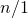=The steepness of the step function for an triangular lattice withcookies in the first row but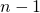in the second (thenin the third,in the fourth, and so on . . .) is:

(average cookies per row)/(distance between rows) =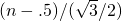=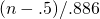With a wide enough cookie sheet, triangular lattices will will out despite having one fewer cookie in every other row when:That means that a triangular lattice with rows of 4-3-4-3-4… will ultimately, once you get above some threshold width, always be more efficient than an square lattice with 4 in every row. But a triangular lattice with rows of 3-2-3-2-3… will ultimately always be less efficient than a square lattice with 3 in every row.

This definitely falls into the category of “curiosity” rather than “useful fact,” though (even more so than the rest of this exercise), since the “thresholds” in question are quite large (91 cookies wide and 27 cookies wide, respectively). So for any sizes of interest, whether a square lattice or a triangular lattice is better depends on the specific width of the pan.

## Equation for the number of cookies that fit on a cookie sheet

First, for the sake of absolute clarity, let’s explicitly define the variables:

• L = cookie sheet length (measured in the units of your choice — inches, cm, furlongs, light-years, etc)
• W = cookie sheet width (measured in the same units as length)
• C = distance between cookie centers (measured in the same units as length and width)

Next, we can simplify to two variables with dimensionless units: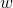= W/C = width measured in cookies= L/C = length measured in cookies

Finally, I should explain that I’ll be using two somewhat uncommon functions:

1. The floor function, written as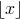when operating on the variable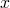, is a mathematical function that rounds a real number down to the nearest integer. (So long as you’re dealing with positive numbers, it’s the same as just dropping whatever is to the right of the decimal point.)
2. The modulo function, written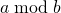for variablesand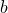, gives the remainder when you divideby. For example,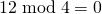(because 4 goes into 12 evenly),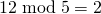, and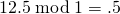.

That done, it’s quite simple to calculate the number of cookies in the largest square lattice that will fit on the cookie sheet. Just round the length and width down to the nearest integer, and multiply them together: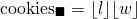Triangular lattices are a bit more complicated, and most easily defined piece-wise:where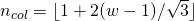is the number of columns that can fit in the width of the sheet.

(This is for a triangular lattice lined up with the length of the cookie sheet, rather the width — the one I dubbed “triangular 1.”)